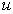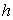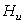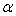# Water Surfaces

If the Groundwater Method = Water Surfaces then you can assign a water surface (e.g. water table, piezo surface) to calculate the pore pressure for a material. Pore pressure is proportional to the vertical distance from the water surface. Water surfaces are created with the Add Water Surface or Add Water by Location options, and may represent a water table, piezo surface or drawdown surface.

In the Material Properties dialog the following parameters can be defined for Water Surfaces.

## Water Surface

The user can assign a Water Surface (e.g. Water Table or Piezo Surface) which corresponds to the material type (soil region) they are defining. Only existing Water Surfaces will appear in the list. The user may also choose None (this is the default selection) if no Water Surface is associated with a given material. If Water Surface = None, pore pressure will be zero for a material.

There are three types of water surface that can be defined - water table, piezo surface or drawdown surface. See the Water Surfaces Overview topic.

## Hu Coefficient

The Hu Coefficient is simply a factor between 0 and 1, by which the VERTICAL distance from a point in the soil (e.g. the center of a column base) to a Water Surface is multiplied to obtain the pressure head. The Hu Coefficient is used to calculate the pore pressure as follows:where:= pore pressure= the Pore Fluid Unit Weight (entered in the Project Settings dialog)= the vertical distance from the base of a column to a Water Surface= the Hu coefficient for the material (user defined or Auto, see below)

• If the distance h is negative, (i.e. Water Surface is below the base of a column) then the pore pressure will be zero or negative, depending on the value of the Negative Pore Pressure Cutoff.
• If a Water Surface is not defined (i.e. does not exist) above a given column, then the safety factor calculation for that particular slip surface will not proceed, and an error message will be written to the file. It is up to the user to ensure that Water Surfaces span all of the required material regions.

There are two ways of defining the Hu Coefficient - Auto or Custom.

## Custom Hu

With the Custom Hu option, the user can enter their own value for Hu. A value between 0 and 1 must be specified. For example:

• Hu = 1 would indicate hydrostatic conditions. This can be used where the Water Surface is horizontal. Where the Water Surface is inclined, setting Hu = 1 will provide a conservative (low) estimate of the safety factor, since in general this will overestimate the true pore pressure. In most cases, the user will simply set Hu = 1, because this represents the worst case scenario (maximum pore pressure).
• Hu = 0 would indicate a dry soil. Pore pressure will be zero. Setting Hu = 0 can be used to turn "off" the pore pressure for a material, although this can also be achieved by setting Water Surface = None.
• Intermediate values of Hu can be used to simulate head loss due to seepage. This would be applicable where the Water Surface is inclined. However, the Auto Hu option, described below, can be used to automatically account for the inclination of the Water Surface.
If you are using Piezometric Surfaces, you should, strictly speaking, use the Custom Hu option, with Hu = 1. This is because a Piezometric Surface is usually a direct representation of the pressure head, for a specific slip surface. However, in Slide3, the user may decide how to apply the Hu Coefficient for any type of Water Surface (Water Table or Piezometric Surface).

## Auto Hu

With the Auto Hu option, Slide3 will automatically calculate a value of Hu, based on the inclination (dip angle) of the Water Surface, above any given point. This is based on the assumption that the equipotential line which passes through the center of a column base, is a straight line between the column base and the Water Surface (strictly applicable for an infinite slope case).

This is illustrated below for the 2-dimensional case. For the actual 3D calculation, the inclination is the 3-dimensional maximum dip angle of the water surface above the center of a column base.

•= the inclination (maximum dip angle) of the Water Surface (above a given point)
•= VERTICAL distance from center of column base to Water Surface